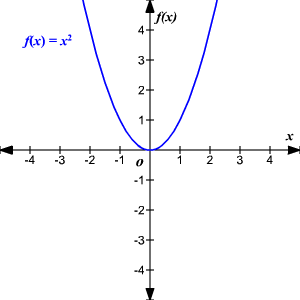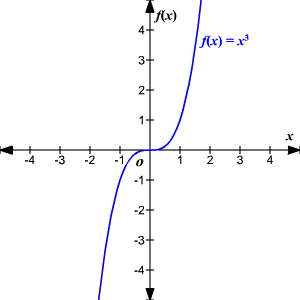# Even/Odd Functions

A function is even if, for each $x$ in the domain of $f$ ,   $f\left(-x\right)=f\left(x\right)$ .  Even functions have reflective symmetry across the $y$ -axis.

Example of an even function:

$\begin{array}{l}f\left(x\right)={x}^{2}\\ f\left(-x\right)={\left(-x\right)}^{2}={x}^{2}=f\left(x\right)\end{array}$A function is odd if, for each $x$ in the domain of $f$$f\left(-x\right)=-f\left(x\right)$ .  Odd functions have $180°$ rotational symmetry about the origin.

Example of an odd function:

$\begin{array}{l}f\left(x\right)={x}^{3}\\ f\left(-x\right)={\left(-x\right)}^{3}=-{x}^{3}=-f\left(x\right)\end{array}$